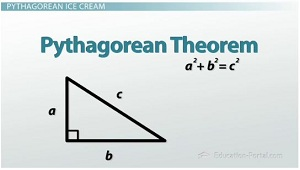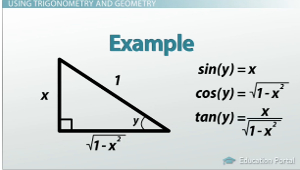# Trigonometry and the Pythagorean Theorem

An error occurred trying to load this video.

Try refreshing the page, or contact customer support.

Coming up next: Radians & Degrees on a Calculator

### You're on a roll. Keep up the good work!

Replay
Your next lesson will play in 10 seconds
• 0:11 SohCahToa and…
• 0:58 Inverse and Reciprocation
• 1:47 Using Trigonometry and…
• 4:07 Lesson Summary
Save Save

Want to watch this again later?

Timeline
Autoplay
Autoplay
Speed Speed Audio mode

#### Recommended Lessons and Courses for You

Lesson Transcript
Instructor: Eric Garneau
Explore how the Pythagorean Theorem can be used in conjunction with trigonometric functions. In this lesson, take an inverse trigonometric function, and define all three sides of a right triangle.

## SohCahToa and the Pythagorean TheoremLet's take one more look at trigonometry. Remember there are two things that you need to keep in mind with respect to trigonometry. You need to remember SohCahToa - sin(theta) equals the opposite over the hypotenuse, cos(theta) equals the adjacent over the hypotenuse, and tan(theta) equals the opposite over the adjacent. So here's our right triangle. We've got theta, the adjacent leg, the opposite leg and the hypotenuse.

The second thing you need to remember is the Pythagorean Theorem. That says that if you have this abc right triangle, a^2 + b^2 = c^2. With those two things, you can do a lot of important calculations in calculus and geometry.

## Inverse and Reciprocation

What are some of the things you might need to know? Well, 1/sin(theta) is known as csc(theta). It's equal to the hypotenuse over the opposite side. We will never write this as sin^-1(theta). Why is that? Well, sin^-1(theta) is really the inverse function of sin(theta); it's not 1/sin(theta). So what this means is that sin^-1(sin(theta)) will give you theta, just like f^-1(f(x)) will give back x. That's the definition of the inverse function here. This also means that if sin(theta) is the opposite over the hypotenuse, the cosecant sin^-1 of the opposite over the hypotenuse is equal to theta.To unlock this lesson you must be a Study.com Member.

### Register to view this lesson

Are you a student or a teacher?

#### See for yourself why 30 million people use Study.com

##### Become a Study.com member and start learning now.
Back
What teachers are saying about Study.com

### Earning College Credit

Did you know… We have over 200 college courses that prepare you to earn credit by exam that is accepted by over 1,500 colleges and universities. You can test out of the first two years of college and save thousands off your degree. Anyone can earn credit-by-exam regardless of age or education level.